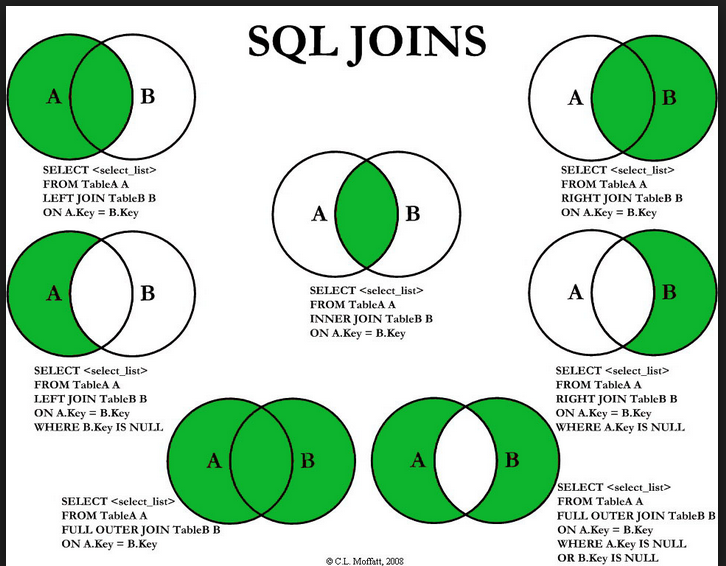## Proc SQL equiv of Merge (If A or B)

I wonder if a full outer join in proc sql is the equivalent of a merge using if (A or B).

DATA All;
MERGE West(IN=A)
East(IN=B);
BY Id;
IF A OR B;
RUN;

3 REPLIES 3

## Re: Proc SQL equiv of Merge (If A or B)

Depends on if you have multiple instances of your BY variables.

There's several good write ups on data step vs sql joins - search on lexjansen.com

http://www2.sas.com/proceedings/sugi30/249-30.pdf

## Re: Proc SQL equiv of Merge (If A or B)

SQL will give you the cartesian product, while the data step will give you as many records for a given by value as were present in one of the input datasets.

example:

dataset a has 2 records for x = 1, while dataset b has 3.

SQL (join on a.x = b.x) will give you six records (all combinations), the data step merge (by x; with if a or b;) 3.

## Re: Proc SQL equiv of Merge (If A or B)

Here is a nice pictorial of SQL joins:Discussion stats
• 3 replies
• 17473 views
• 0 likes
• 4 in conversation